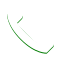##### Our Key Values
=-=-=-=-=-=-=-=-=-=
English, Maths, Science & Malay
PSLE, NT, N & O Level
=-=-=-=-=-=-=-=-=-=Based on Latest MOE SyllabusProgress MonitoredPictorial Concept ApproachDevelop Inquiry SkillsIncorporates Problem Solving SkillsSmall Group (8 Students : 1 Tutor)Nurture Self MotivationWork with Your Child Ability Level80% of our Students Improved After 6 Months They Joined Us

Model Approach to Problem-Solving

Subscribe now to watch video explanation of Model Approach to Problem Solving.

1. Whole Numbers
2. Division
3. Multiplication
4. Length and Mass
5. Volume & Capacity
6. Money
7. Fraction
8. Area & Perimeter

\$97 / year

Model Approach to Problem-Solving

Subscribe now to watch video explanation of Model Approach to Problem Solving.

1. Whole Numbers
2. Multiplication & Division
3. Fraction
4. Decimal

\$97 / year

Model Approach to Problem-Solving

Subscribe now to watch video explanation of Model Approach to Problem Solving.

1. Whole Numbers
2. Fraction
3. Ratio
4. Rate
5. Average
6. Decimal
7. Percentage
8. Volume

\$97 / year

Model Approach to Problem-Solving

Subscribe now to watch video explanation of Model Approach to Problem Solving.

1. Algebra
2. Fraction
3. Ratio
4. Percentage

\$97 / year

Model Approach to Problem-Solving

Subscribe now to watch video explanation of Model Approach to Problem Solving.

1. Speed
2. Circles
3. Pie Chart
4. Area & Perimeter
5. Volume

\$97 / yearNeed Help?+6584078216